## 2. 1. 2 Errors of a Numerically Discrete System

When performing numerical operations on one input variable, the error can be estimated a in straight forward manner. In contrast, the handling of a system of equations is more difficult to estimate. Methods which are used to solve algebraic problems have to be considered with respect to their behavior when dealing with truncated or rounded numerical values. For the following considerations the equation system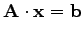(2.1)

is used, where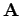is the matrix,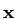is the solution vector and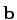is the right-hand-side vector. The typical measure to describe an equation system is the so-called condition number .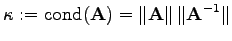(2.2)

This condition number states the deviation of the solution vector of a linear equation system measured by its norm with respect to the right hand side vector of the equation system .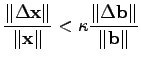(2.3)

Such an estimation is useful, if the right hand side is added a numerical error, e.g. noise caused by discretization. If numerical noise is added to the matrix, which is typically the case when material parameters or geometrical coefficients for discretization schemes have to be determined via floating point computations including all occurring errors, another consideration can be used :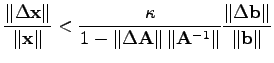(2.4)

Errors of this kind are considered in the numerical treatment of algebraic methods as shown in detail in . In the following considerations, the numerical error is not further considered, because the main focus of this work is the specification of formulae rather than the numerical treatment or the solution of special problems.

Michael 2008-01-16